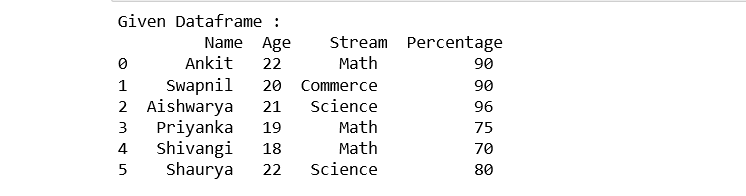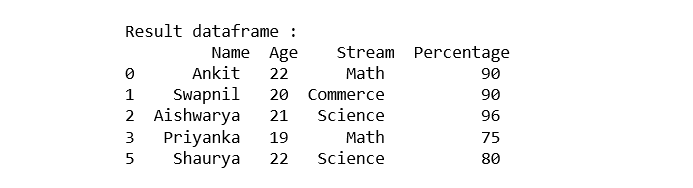# Ways to filter Pandas DataFrame by column values

• Last Updated : 01 Oct, 2020

In this post, we will see different ways to filter Pandas Dataframe by column values. First, Let’s create a Dataframe:

## Python3

 `# importing pandas ``import` `pandas as pd ``   ` `# declare a dictionary``record ``=` `{ `` ` ` ``'Name'` `: [``'Ankit'``, ``'Swapnil'``, ``'Aishwarya'``, ``          ``'Priyanka'``, ``'Shivangi'``, ``'Shaurya'` `],``   ` ` ``'Age'` `: [``22``, ``20``, ``21``, ``19``, ``18``, ``22``], ``   ` ` ``'Stream'` `: [``'Math'``, ``'Commerce'``, ``'Science'``, ``            ``'Math'``, ``'Math'``, ``'Science'``], ``   ` ` ``'Percentage'` `: [``90``, ``90``, ``96``, ``75``, ``70``, ``80``] } ``   ` `# create a dataframe ``dataframe ``=` `pd.DataFrame(record,``                         ``columns ``=` `[``'Name'``, ``'Age'``, ``                                    ``'Stream'``, ``'Percentage'``]) ``# show the Dataframe``print``(``"Given Dataframe :\n"``, dataframe)`

Output:Method 1: Selecting rows of Pandas Dataframe based on particular column value using ‘>’, ‘=’, ‘=’, ‘<=’, ‘!=’ operator.

Example 1: Selecting all the rows from the given Dataframe in which ‘Percentage’ is greater than 75 using [ ].

## Python3

 `# selecting rows based on condition ``rslt_df ``=` `dataframe[dataframe[``'Percentage'``] > ``70``] ``   ` `print``(``'\nResult dataframe :\n'``, rslt_df)`

Output:Example 2: Selecting all the rows from the given Dataframe in which ‘Percentage’ is greater than 70 using loc[ ]

## Python3

 `# selecting rows based on condition ``rslt_df ``=` `dataframe.loc[dataframe[``'Percentage'``] > ``70``] ``   ` `print``(``'\nResult dataframe :\n'``, ``      ``rslt_df)`

Output:Method 2: Selecting those rows of Pandas Dataframe whose column value is present in the list using isin() method of the dataframe.

Example 1: Selecting all the rows from the given dataframe in which ‘Stream’ is present in the options list using [ ].

## Python3

 `options ``=` `[``'Science'``, ``'Commerce'``] ``   ` `# selecting rows based on condition ``rslt_df ``=` `dataframe[dataframe[``'Stream'``].isin(options)] ``   ` `print``(``'\nResult dataframe :\n'``,``      ``rslt_df)`

Output:Example 2: Selecting all the rows from the given dataframe in which ‘Stream’ is present in the options list using loc[ ].

## Python

 `options ``=` `[``'Science'``, ``'Commerce'``] ``   ` `# selecting rows based on condition ``rslt_df ``=` `dataframe.loc[dataframe[``'Stream'``].isin(options)] ``   ` `print``(``'\nResult dataframe :\n'``, ``      ``rslt_df)`

Output:Method 3: Selecting rows of  Pandas Dataframe based on multiple column conditions using ‘&’ operator.

Example1: Selecting all the rows from the given Dataframe in which ‘Age’ is equal to 22 and ‘Stream’ is present in the options list using [ ].

## Python3

 `options ``=` `[``'Commerce'` `,``'Science'``] ``   ` `# selecting rows based on condition ``rslt_df ``=` `dataframe[(dataframe[``'Age'``] ``=``=` `22``) & ``          ``dataframe[``'Stream'``].isin(options)] ``   ` `print``(``'\nResult dataframe :\n'``,``      ``rslt_df)`

Output:Example 2: Selecting all the rows from the given Dataframe in which ‘Age’ is equal to 22 and ‘Stream’ is present in the options list using loc[ ].

## Python3

 `options ``=` `[``'Commerce'``, ``'Science'``] ``  ` `# selecting rows based on condition ``rslt_df ``=` `dataframe.loc[(dataframe[``'Age'``] ``=``=` `22``) & ``              ``dataframe[``'Stream'``].isin(options)] ``   ` `print``(``'\nResult dataframe :\n'``,``      ``rslt_df)`

Output:My Personal Notes arrow_drop_up